# Volume 21, 2015, Number 3

Volume 21Number 1Number 2 ▷ Number 3 ▷ Number 4

“Theory of Numbers” of a complete region
Original research paper. Pages 1—21
Ranjit Biswas
Full paper (PDF, 2730 Kb) | Abstract

This is sequel to our previous work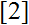,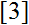,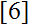on region algebra. An important contribution inis that the minimum platform required and being used so far by the world for practicing elementary algebra is unearthed and uniquely identified which is not group, ring, field, module, linear space, algebra over a field, associative algebra over a field, division algebra, or any existing algebraic system in general, but ‘Region Algebra’. The properties of region algebra are interesting as this is the minimal algebra which justifies free and fluent practice of elementary as well as higher algebra. This important identification was missing so far in any past literature of algebra or mathematics, and thus it is surely a unique algebra of absolute integrated nature. A new theory called by “Theory of Objects” and as a special case of it the classical “Theory of Numbers” were also studied in,. In this paper we say that every complete region A has its own ‘Theory of Numbers’ called by ‘Theory of A-numbers’, where the classical ‘Theory of Numbers’ is the ‘Theory of RR-numbers’ corresponding to the particular complete region RR. For the sake of presentation and to avoid any confusion we consider three theories here but finally we arrive at a unified unique theory at the end. The three theories designated in this paper are: Theory-1 (Theory of Numbers) which is exactly the existing “Theory of Numbers” in the literature (on real numbers and complex numbers); Theory-2 (Theory of Objects) which is about combinatorics on Region Algebra, about prime objects and composite objects, about a new ‘Theory of Numbers’ corresponding to every complete region (viz. the ‘Theory of A-numbers’ is corresponding to the complete region A, etc.); and Theory-3 (Theory of RR-numbers) which is all about the “Theory of RR-numbers”, a particular case of the “Theory of A-numbers” of Theory-2 where the region A is the complete region RR. In fact Theory-1 happens to be a special case of Theory-3, but initiating of Theory-3 done by the author is not with the purpose of ‘Making a generalization of the Theory-1’. It may also be noted that the Theory-3 is a special case of “Theory of A-numbers” where “Theory of A-numbers” is derived from the “Theory of Objects” of Theory-2. The “Theory of Objects” also induces a new field called by “Object Geometry” of a complete region, being a generalization of our rich classical geometry of the existing notion. It is claimed that the “Theory of Objects” will play a huge role to the Number Theorists in a new direction.

A variant of Waring’s problem
Original research paper. Pages 22—26
Abdullah N. Arslan
Full paper (PDF, 155 Kb) | Abstract

We introduce a variant of Waring’s problem. For a given positive integer k, consider the problem of writing any given positive integer N as the sum of the kth powers of consecutive integers starting at 1 using each of these kth powers (summands) exactly once, and repeating some of these summands as necessary. Let Ck denote the total number of such repeats. Determine minimum Ck for positive integers k required to write all positive integers using the kth powers of consecutive integers as described. We show that Ck ≤ g(k), where g(k) is the usual notation in Waring’s problem, the least number of non-negative kth powers sufficient to represent all positive integers. This result implies that for any given positive integer k, every positive integer N can be expressed as a linear combination of the kth powers of consecutive integers with positive integer coefficients that satisfy certain inequalities. Another implication is that for all positive integers N; n′; and k, the equation N = Σi = 1n′ikxi has at least one solution (x1, x2, …, xn′) in nonnegative integers if Σi = 1n′ik ≥ N.

A Möbius arithmetic incidence function
Original research paper. Pages 27—34
Emil Daniel Schwab and Gabriela Schwab
Full paper (PDF, 171 Kb) | Abstract

The aim of this note is to study a non-standard right cancellative and half-factorial Möbius monoid, and to compute its Möbius function

Short remark on intuitionistic fuzziness and square-free numbers
Original research paper. Pages 35—37
Krassimir T. Atanassov
Full paper (PDF, 129 Kb) | Abstract

Two examples of possible relationships between elements of number theory and intuitionistic fuzzy set theory are given.

Improving Riemann prime counting
Original research paper. Pages 38—44
Michel Planat and Patrick Solé
Full paper (PDF, 201 Kb) | Abstract

Prime number theorem asserts that (at large x) the prime counting function π(x) is approximately the logarithmic integral li(x). In the intermediate range, Riemann prime counting function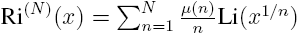deviates from π(x) by the asymptotically vanishing sum ΣρRi(xρ) depending on the critical zeros ρ of the Riemann zeta function ζ(s). We find a fit π(x) ≈ Ri(3){ψ(x)} (with three to four new exact digits compared to li(x)) by making use of the Von Mangoldt explicit formula for the Chebyshev function ψ(x). Another equivalent fit makes use of the Gram formula with the variable ψ(x). Doing so, we evaluate π(x) in the range x = 10i,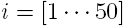with the help of the first 2×106 Riemann zeros ρ. A few remarks related to Riemann hypothesis (RH) are given in this context.

On some Pascal’s like triangles. Part 11
Original research paper. Pages 45—55
Krassimir T. Atanassov
Full paper (PDF, 213 Kb) | Abstract

In a series of papers, Pascal’s like triangles with different forms have been described. Here, new types of triangles is discussed. In the formula for their generation, operation summation is replaced, respectively, by operations multiplication and exponentiation. Some of their properties are studied. The general case is discussed.

Tridiagonal matrices related to subsequences of balancing and Lucas-balancing numbers
Original research paper. Pages 56—63
Prasanta K Ray and Gopal K Panda
Full paper (PDF, 176 Kb) | Abstract

It is well known that balancing and Lucas-balancing numbers are expressed as determinants of suitable tridiagonal matrices. The aim of this paper is to express certain subsequences of balancing and Lucas-balancing numbers in terms of determinants of tridiagonal matrices. Using these tridiagonal matrices, a factorization of the balancing numbers is also derived

Pell and Lucas primes
Original research paper. Pages 64—69
J. V. Leyendekkers and A. G. Shannon
Full paper (PDF, 95 Kb) | Abstract

The structures of Pell and Lucas numbers, Pp and Lp with prime subscripts are compared in relation to the function (Kp ± 1) and for factors of the form (kp ± 1). It is found that digit sums give some guides to primality

Trigonometric Pseudo Fibonacci Sequence
Original research paper. Pages 70—76
C. N. Phadte and S. P. Pethe
Full paper (PDF, 171 Kb) | Abstract

In this paper, we establish some results about second order non homogeneous recurrence relation containing extended trignometric function. Earlier {4}, we proved some properties of recurrence relation
gn+2 = gn+1 + gn + Atn, n = 0, 1, … with g0 = 0, g1 = 1; where both A ≠ 0 and t ≠ 0, and also t ≠ α, β where α, β are the roots of x2 − x − 1 = 0.
Using the properties of generalised circular functions and Elmore’s method, we define a new sequence {Hn} which is the extension of Pseudo Fibonacci Sequence, given by recurrence relation
Hn+2 = pHn+1 − qHn + RtnNr,0(t*x),
where Nr,0(t*x) is extended circular function.
We state and prove some properties for this extended Pseudo Fibonacci Sequence {Hn} .

Congruence properties of some partition functions
Original research paper. Pages 77—79
Manvendra Tamba
Full paper (PDF, 102 Kb) | Abstract

Let M(n) = (N3(n)/48), where N3(n) denotes the number of ways in which n can be written as sum of three squares. We study the congruence properties of some partition
functions in relation to M(16n + 14).

On the density of ranges of generalized divisor functions
Original research paper. Pages 80—87
Colin Defant
Full paper (PDF, 173 Kb) | Abstract

The range of the divisor function σ−1 is dense in the interval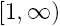. However, although the range of the function σ−2 is a subset of the interval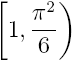, we will see that the range of σ−2 is not dense in. We begin by generalizing the divisor functions to a class of functions σt for all real t. We then define a constant η ≈ 1.8877909 and show that if r ∈ (1, ∞), then the range of the function σ−r is dense in the interval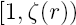if and only if r ≤ η. We end with an open problem.

Volume 21Number 1Number 2 ▷ Number 3 ▷ Number 4